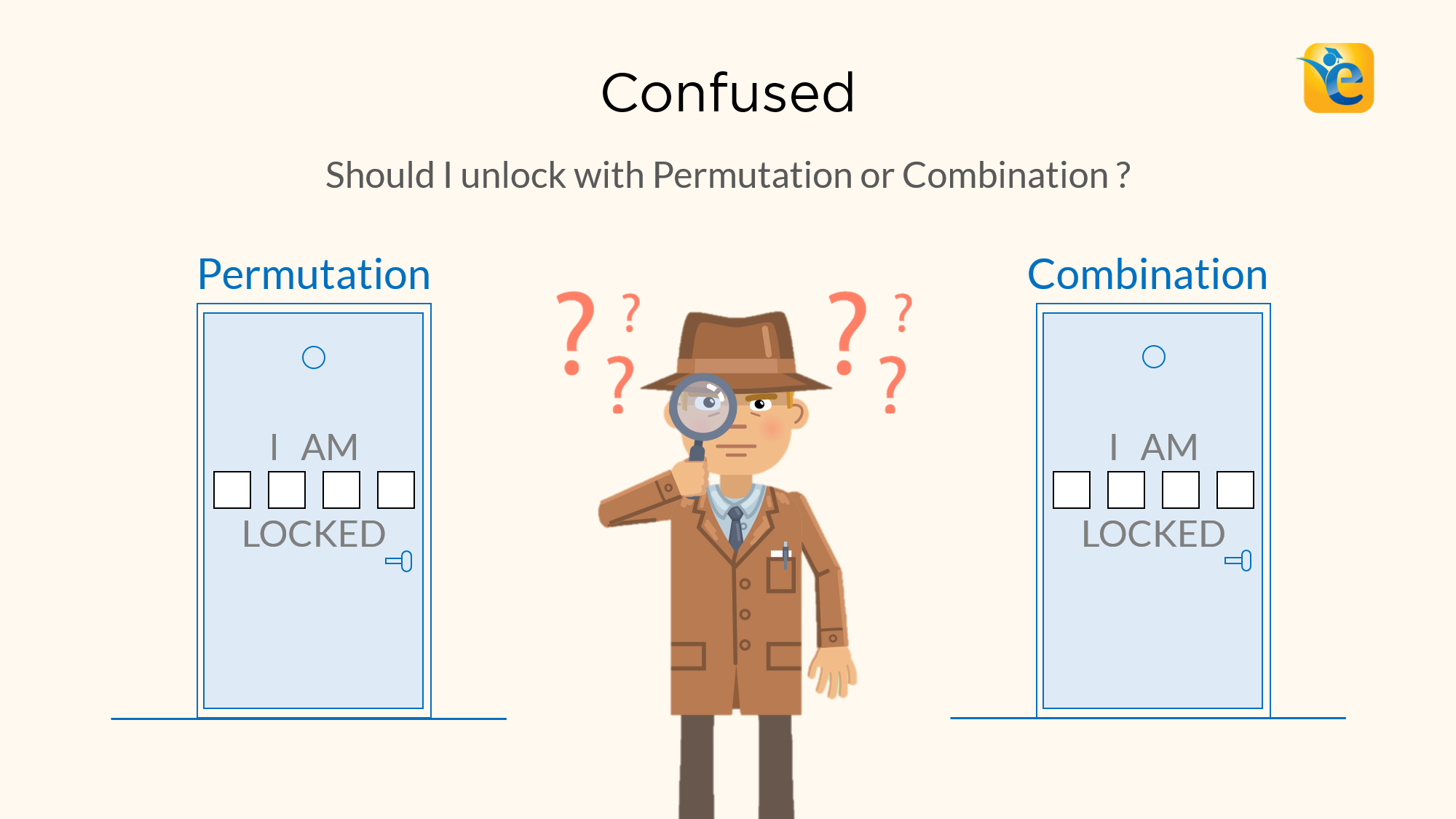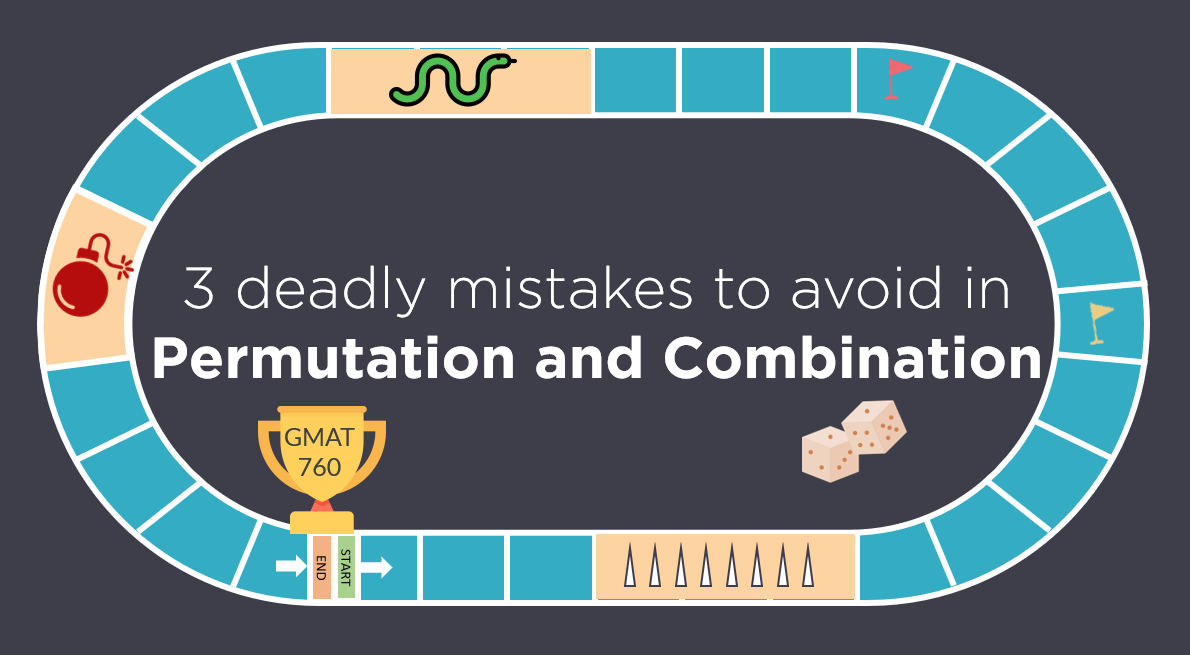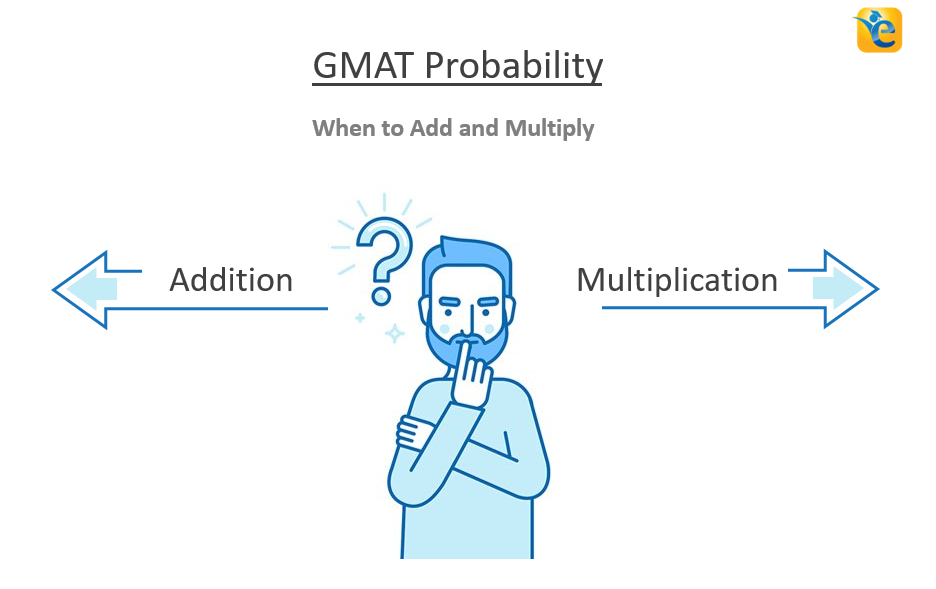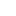Payal Tandon
Co-founder, e-GMAT
Welcome to e-GMAT Support!
I am Payal, Co-Founder of e-GMAT.
Feel free to ask any Query.We will be contacting you soon on# Difference between Permutation and Combination explained with examples

Permutations and Combinations is a topic full of conundrums. The biggest one is, understanding the difference between permutation and combination. Should I solve this...# Permutation and Combination | Avoid these 3 Mistakes | GMAT Quant

Like any GMAT Quant topic, Permutation and Combination has its own traps. Most students fall in these traps and ultimately, are not able to secure their target GMAT score....# GMAT Permutation and Combination | When to Add and Multiply

Are you always confused whether to add or multiply the cases while solving GMAT permutation and combination questions? Do you often get questions incorrect simply because...# Difference between Permutation and Combination explained with examples

Permutations and Combinations is a topic full of conundrums. The biggest one is, understanding the difference between permutation and combination....# Permutation and Combination | Avoid these 3 Mistakes | GMAT Quant

Like any GMAT Quant topic, Permutation and Combination has its own traps. Most students fall in these traps and ultimately, are not able to secure...# GMAT Permutation and Combination | When to Add and Multiply

Are you always confused whether to add or multiply the cases while solving GMAT permutation and combination questions? Do you often get questions...

# GMAT Problem Solving – 3 mistakes you must avoid in Time and Work questions

GMAT problem solving questions on Time and Work can be solved easily and quickly provided you do not make mistakes while solving them. In the last...

# GMAT Word Problems | Application of Average Speed in Time – Distance Problems

GMAT Word problems on Time, and Speed, and Distance are based on the simple formula of  D = S x T, where D is the distance, S is speed and T is...

# More articles

## Difference between Permutation and Combination explained with examples

A 8 min read Permutations and Combinations is a topic full of conundrums. The biggest one is,...

## Permutation and Combination | Avoid these 3 Mistakes | GMAT Quant

A 12 min read Like any GMAT Quant topic, Permutation and Combination has its own traps. Most...

## GMAT Permutation and Combination | When to Add and Multiply

A 10 min read Are you always confused whether to add or multiply the cases while solving GMAT...

## GMAT Problem Solving – 3 mistakes you must avoid in Time and Work questions

A 10 min read GMAT problem solving questions on Time and Work can be solved easily and quickly provided you do not make mistakes while solving them. In the last article, we compared the conventional methods of solving Time and...

## GMAT Word Problems | Application of Average Speed in Time – Distance Problems

A 12 min read GMAT Word problems on Time, and Speed, and Distance are based on the simple formula of  D = S x T, where D is the distance, S is speed and T is time. These GMAT word problems might look easy at first but if...

## How to quickly solve GMAT Quant Questions on Time and Work

A 13 min read Time and Work problems are the low-hanging fruit in the world of GMAT Quant...

## If Car A took n hours to travel 2 miles and Car B took m hours to travel 3 miles, which of the following expresses the time it would take Car C, traveling at the average (arithmetic mean) of those rates, to travel 5 miles? – OGQR 2020 Question #79 with Solution

A < 1 min read OGQR 2020: Question No. 79 If Car A took n hours to travel 2 miles and Car B took m hours to travel 3 miles, which of the following expresses the time it would take Car C, traveling at the average (arithmetic...

## P is a particle on the circle shown above. What is the length of the path travelled by P in one complete revolution around the circle? – OGQR 2020 Question #212 with Solution

A 2 min read OGQR 2020: Question No. 212 P is a particle on the circle shown above. What is the length of the path traveled by P in one complete revolution around the circle? The diameter of the circle is...

## Carl averaged 2m miles per hour on a trip that took him h hours. If Ruth made the same trip in h hours, what was her average speed in miles per hour? – OGQR 2020 Question #82 with Solution

A < 1 min read OGQR 2020: Question No. 82 Carl averaged 2m miles per hour on a trip that took him h hours. If Ruth made the same trip in (2/3)h hours, what was her average speed in miles per hour? Source OGQR 2020 Type...###### Don't limit yourself!# Achieve 740+on the GMAT in 30 days!Sign up for our free trial and get:400+ Practice questions with detailed solutions10+ hours of AI-driven video lessonsAdaptive mock test with ESR+ analysis